# Bayesian Learning Chapter 20 1 20 4 Some

• Slides: 16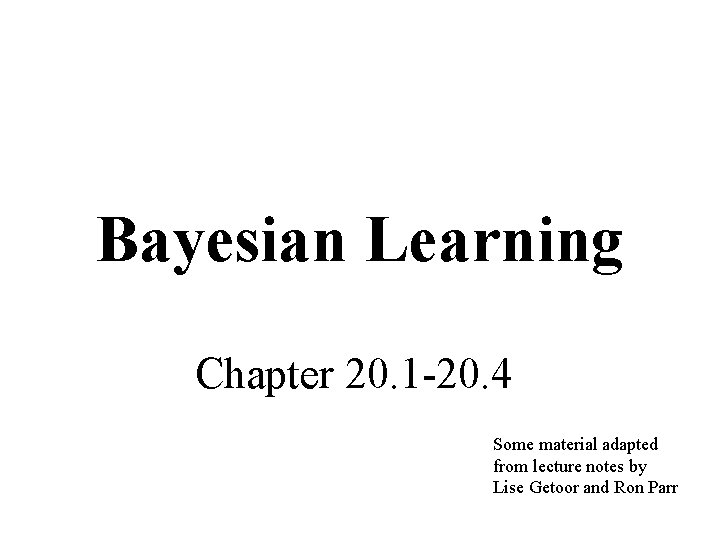Bayesian Learning Chapter 20. 1 -20. 4 Some material adapted from lecture notes by Lise Getoor and Ron Parr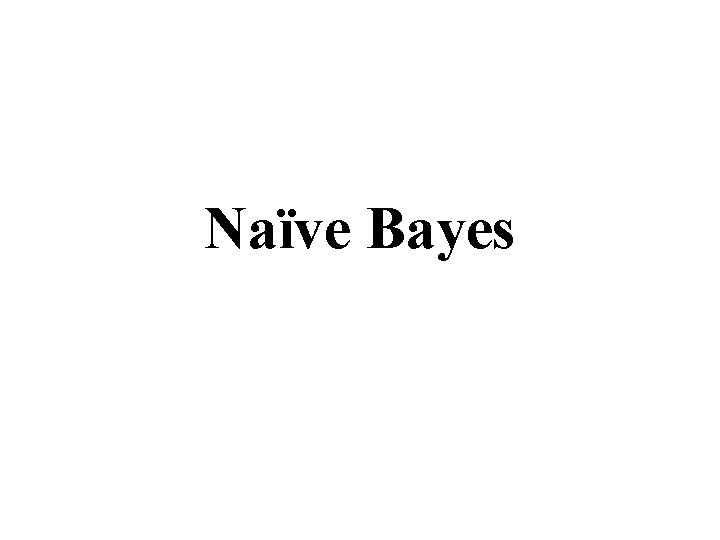Naïve Bayes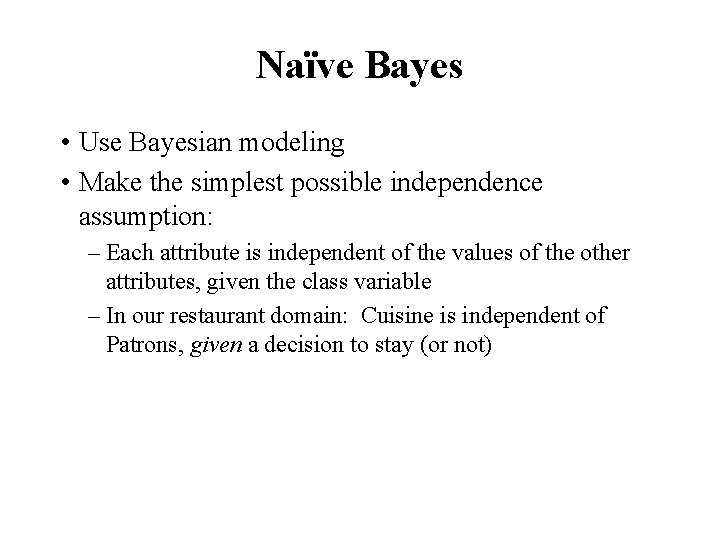Naïve Bayes • Use Bayesian modeling • Make the simplest possible independence assumption: – Each attribute is independent of the values of the other attributes, given the class variable – In our restaurant domain: Cuisine is independent of Patrons, given a decision to stay (or not)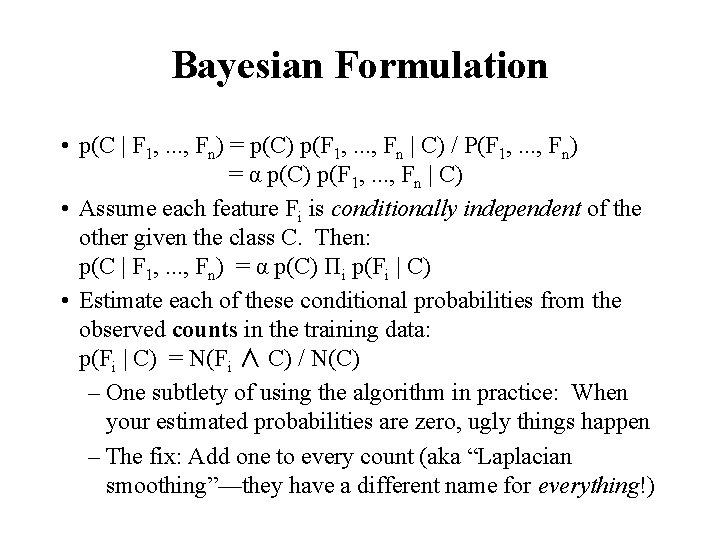Bayesian Formulation • p(C | F 1, . . . , Fn) = p(C) p(F 1, . . . , Fn | C) / P(F 1, . . . , Fn) = α p(C) p(F 1, . . . , Fn | C) • Assume each feature Fi is conditionally independent of the other given the class C. Then: p(C | F 1, . . . , Fn) = α p(C) Πi p(Fi | C) • Estimate each of these conditional probabilities from the observed counts in the training data: p(Fi | C) = N(Fi ∧ C) / N(C) – One subtlety of using the algorithm in practice: When your estimated probabilities are zero, ugly things happen – The fix: Add one to every count (aka “Laplacian smoothing”—they have a different name for everything!)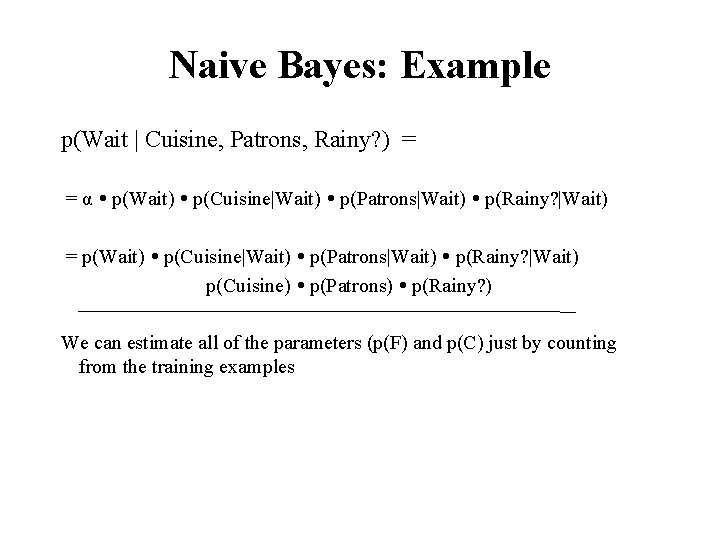Naive Bayes: Example p(Wait | Cuisine, Patrons, Rainy? ) = = α p(Wait) p(Cuisine|Wait) p(Patrons|Wait) p(Rainy? |Wait) = p(Wait) p(Cuisine|Wait) p(Patrons|Wait) p(Rainy? |Wait) p(Cuisine) p(Patrons) p(Rainy? ) We can estimate all of the parameters (p(F) and p(C) just by counting from the training examples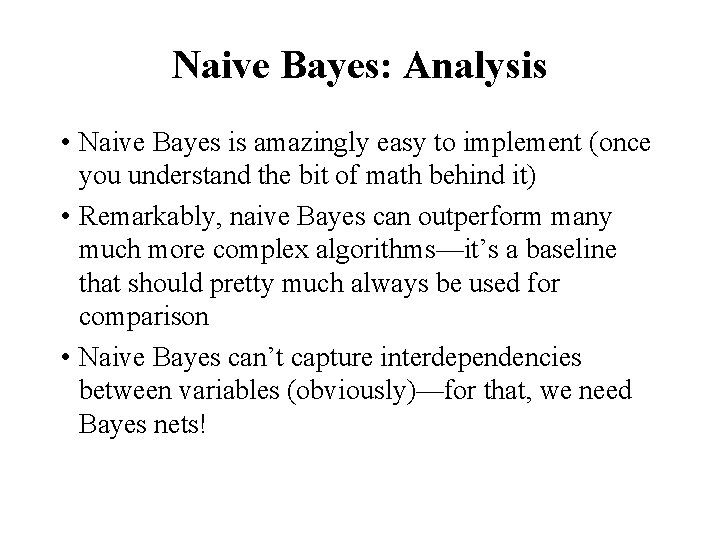Naive Bayes: Analysis • Naive Bayes is amazingly easy to implement (once you understand the bit of math behind it) • Remarkably, naive Bayes can outperform many much more complex algorithms—it’s a baseline that should pretty much always be used for comparison • Naive Bayes can’t capture interdependencies between variables (obviously)—for that, we need Bayes nets!Learning Bayesian NetworksLearning Bayesian networks • Given training set • Find B that best matches D – model selection – parameter estimation B Inducer E A C Data D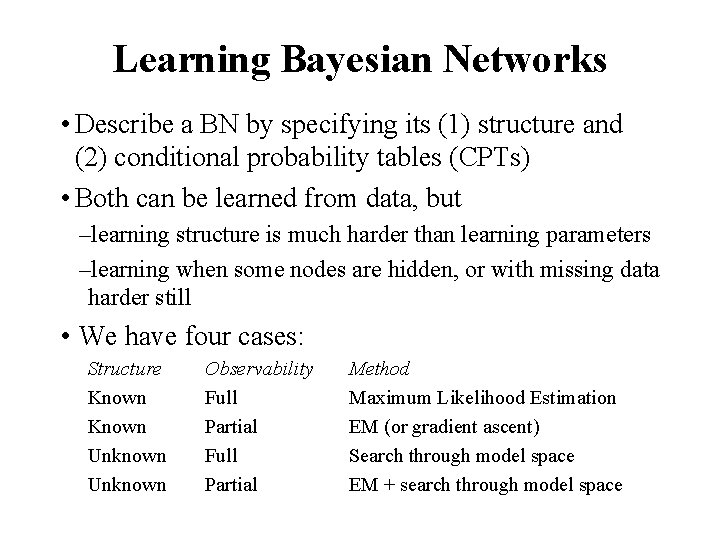Learning Bayesian Networks • Describe a BN by specifying its (1) structure and (2) conditional probability tables (CPTs) • Both can be learned from data, but –learning structure is much harder than learning parameters –learning when some nodes are hidden, or with missing data harder still • We have four cases: Structure Known Unknown Observability Full Partial Method Maximum Likelihood Estimation EM (or gradient ascent) Search through model space EM + search through model spaceParameter estimation • Assume known structure • Goal: estimate BN parameters q – entries in local probability models, P(X | Parents(X)) • A parameterization q is good if it is likely to generate the observed data: i. i. d. samples • Maximum Likelihood Estimation (MLE) Principle: Choose q* so as to maximize L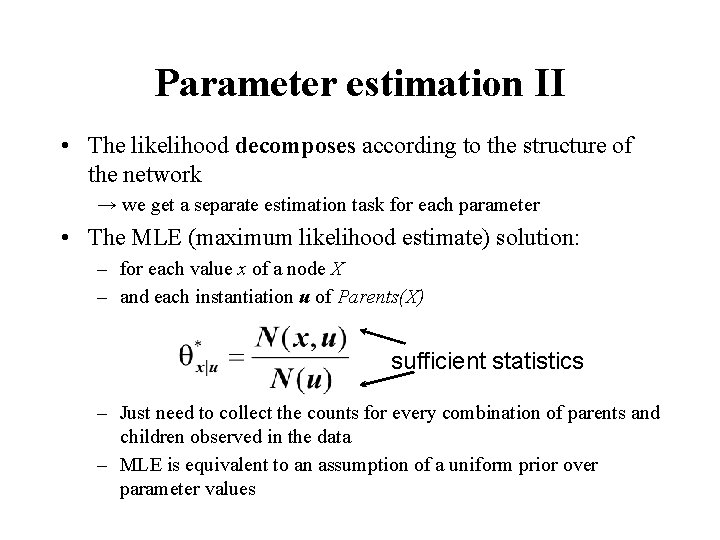Parameter estimation II • The likelihood decomposes according to the structure of the network → we get a separate estimation task for each parameter • The MLE (maximum likelihood estimate) solution: – for each value x of a node X – and each instantiation u of Parents(X) sufficient statistics – Just need to collect the counts for every combination of parents and children observed in the data – MLE is equivalent to an assumption of a uniform prior over parameter values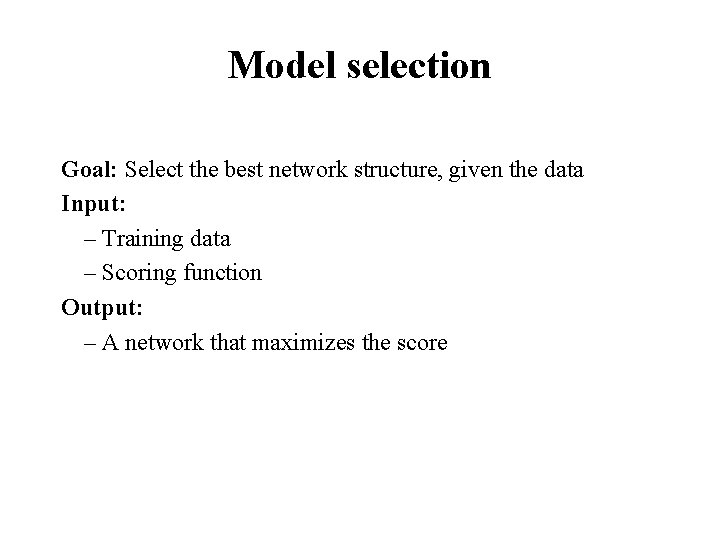Model selection Goal: Select the best network structure, given the data Input: – Training data – Scoring function Output: – A network that maximizes the score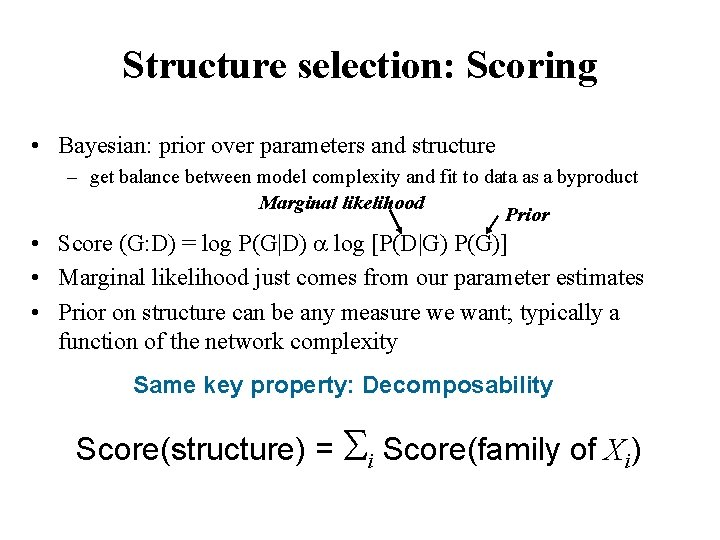Structure selection: Scoring • Bayesian: prior over parameters and structure – get balance between model complexity and fit to data as a byproduct Marginal likelihood Prior • Score (G: D) = log P(G|D) log [P(D|G) P(G)] • Marginal likelihood just comes from our parameter estimates • Prior on structure can be any measure we want; typically a function of the network complexity Same key property: Decomposability Score(structure) = Si Score(family of Xi)Heuristic search B B E A A E se ) r E e , v A e( Re r o Δsc E B C C E Add (C) re o c Δs De let e. E Δs A cor e(A ) E A C B E A A C C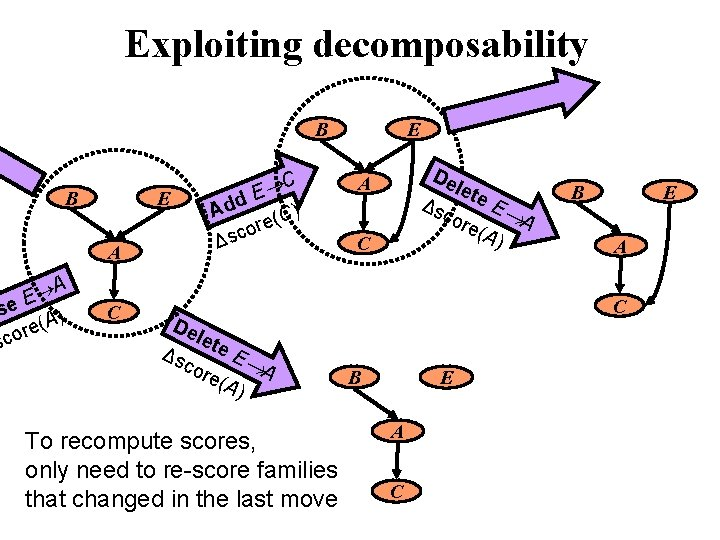Exploiting decomposability B B E A A E se ) A ( e r sco C C E Add (C) re o c Δs De let e. E Δs A cor e(A ) To recompute scores, only need to re-score families that changed in the last move E De let e. E Δs A cor e(A ) A C B E A CVariations on a theme • Known structure, fully observable: only need to do parameter estimation • Unknown structure, fully observable: do heuristic search through structure space, then parameter estimation • Known structure, missing values: use expectation maximization (EM) to estimate parameters • Known structure, hidden variables: apply adaptive probabilistic network (APN) techniques • Unknown structure, hidden variables: too hard to solve!